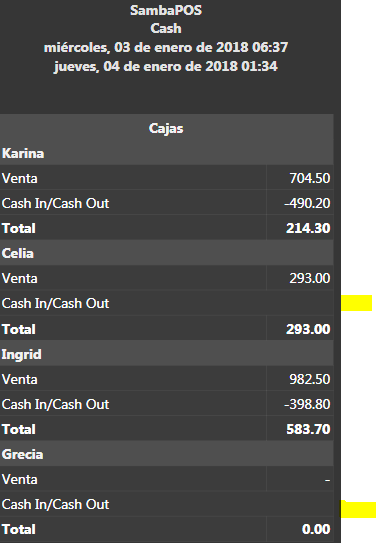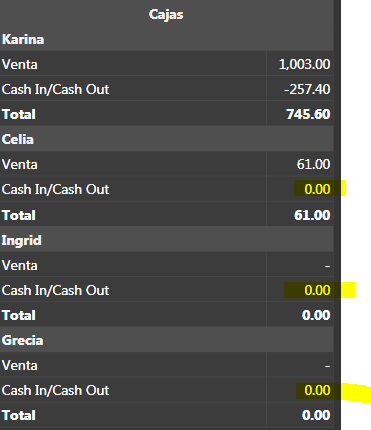SQL IIF syntax for report

Hello there,

I try to make my report better.
When using:

• {REPORT xxx}; Null numbers or empty result are shown as ‘-’.
• F(TNxxx); Null numbers are shown as 0.00
• SQL result depends but shows nothing

Which for printed reports is bad:
If you have 2 columns and 1 of the 2 columns is “empty/nothing”, the layout varies:

So I try to put “-” if the result of SQL is empty or zero.
Without success …

[Cajas:4, 1, 1]
{REPORT SQL DETAILS:
SELECT
‘Cash In/Cash Out’ as CashIN
,IIF(sum([Debit]+[Credit]) = 0,‘test’,sum([Debit]+[Credit]*-1)) as [txTotal]
FROM [AccountTransactionValues]
WHERE …}

IIF works apparently but I cannot figure out the condition of ‘IsEmpty/Null/zero’ …
I tried =
‘’
NULL
0
‘0’
“0”
<> 0

Show your template I’m sure we can format it not to do that.

Visual is good … (except I want ‘-’ instead of blank)My template:

@ Karina,Celia,Ingrid,Grecia,
[Cajas:4, 1]
>>\$1
Venta|{REPORT PAYMENT TOTAL:(PT=Cash) AND (PU=\$1) and (TY=Ticket)}
{REPORT SQL DETAILS:
SELECT
‘Cash In/Cash Out’ as CashIN
,IIF(sum([Debit]+[Credit]) > 0,’-’,sum([Debit]+[Credit]*-1)) as [txTotal]
FROM [AccountTransactionValues]
WHERE [Name] LIKE ‘%\$1’+’%’ AND [AccountId]=5
AND SUBSTRING(REPLACE(CONVERT(varchar(50),[Date],126),‘T’,’ ‘),1,16) >= SUBSTRING(REPLACE(CONVERT(varchar(50),’{Start}’,126),‘T’,’ ‘),1,16)
AND SUBSTRING(REPLACE(CONVERT(varchar(50),[Date],126),‘T’,’ ‘),1,16) <= SUBSTRING(REPLACE(CONVERT(varchar(50),’{End}’,126),‘T’,’ ‘),1,16)
:F.CashIN,F.txTotal
}
>Total|[=F(TN(’{REPORT PAYMENT TOTAL:(PT=Cash) AND (PU=\$1) and (TY=Ticket)}’)+TN(’{REPORT SQL DETAILS: SELECT sum([Debit]+[Credit]*-1) as [txTotal] FROM [AccountTransactionValues] WHERE [Name] LIKE ‘%\$1’+’%’ AND [AccountId]=5 AND SUBSTRING(REPLACE(CONVERT(varchar(50),[Date],126),‘T’,’ ‘),1,16) >= SUBSTRING(REPLACE(CONVERT(varchar(50),’{Start}’,126),‘T’,’ ‘),1,16) AND SUBSTRING(REPLACE(CONVERT(varchar(50),[Date],126),‘T’,’ ‘),1,16) <= SUBSTRING(REPLACE(CONVERT(varchar(50),’{End}’,126),‘T’,’ ‘),1,16) :F.txTotal }’))]

Better said, how can I do something like that:

{REPORT SQL DETAILS:
SELECT
IIF([Debit] = 0,’-’,[Debit]) as [txDebit]
FROM [AccountTransactionValues]
:TxDebit}

What is the type of Debit in AccountTransactionValues?

Ok I understand, If there is no transaction, Debit does not exist, SQL reports 0 row, so we will not enter in the IF test clause, so no “-”.

I have to replace “empty” outside of the {REPORT}, so:

Is now:

@ Karina,Celia,Ingrid,Grecia,
[Cajas:4, 1]
>>\$1|
Venta|{REPORT PAYMENT TOTAL:(PT=Cash) AND (PU=\$1) and (TY=Ticket)}
Cash In/Cash Out|[=F(TN(’{REPORT SQL DETAILS:SELECT sum([Debit]+[Credit]*-1) as [txTotal] FROM [AccountTransactionValues] WHERE [Name] LIKE ‘%\$1’+’%’ AND [AccountId]=5 AND SUBSTRING(REPLACE(CONVERT(varchar(50),[Date],126),‘T’,’ ‘),1,16) >= SUBSTRING(REPLACE(CONVERT(varchar(50),’{Start}’,126),‘T’,’ ‘),1,16) AND SUBSTRING(REPLACE(CONVERT(varchar(50),[Date],126),‘T’,’ ‘),1,16) <= SUBSTRING(REPLACE(CONVERT(varchar(50),’{End}’,126),‘T’,’ ‘),1,16):F.txTotal}’))]
>Total|[=F(TN(’{REPORT PAYMENT TOTAL:(PT=Cash) AND (PU=\$1) and (TY=Ticket)}’)+TN(’{REPORT SQL DETAILS: SELECT sum([Debit]+[Credit]*-1) as [txTotal] FROM [AccountTransactionValues] WHERE [Name] LIKE ‘%\$1’+’%’ AND [AccountId]=5 AND SUBSTRING(REPLACE(CONVERT(varchar(50),[Date],126),‘T’,’ ‘),1,16) >= SUBSTRING(REPLACE(CONVERT(varchar(50),’{Start}’,126),‘T’,’ ‘),1,16) AND SUBSTRING(REPLACE(CONVERT(varchar(50),[Date],126),‘T’,’ ‘),1,16) <= SUBSTRING(REPLACE(CONVERT(varchar(50),’{End}’,126),‘T’,’ ‘),1,16) :F.txTotal }’))]

I don’t have my ‘-’, but at least I have 0.00 instead of empty, printed report is easier to read.2 Likes

It seems that you’ve got your problem under control, but just for future reference, to see if a value is null in SQL you can use the “[rowName] IS NULL”.